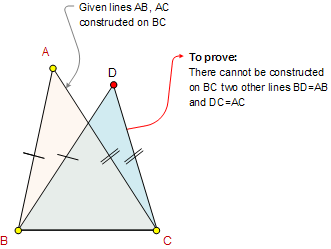Euclid's Elements Book I, Proposition 7:
Given two straight lines constructed on a straight line (from its extremities) and meeting in a point, there cannot be constructed on the same straight line (from its extremities), and on the same side of it, two other straight lines meeting in another point and equal to the former two respectively, namely each to that has the same extremity with it.

Given two straight lines AB, AC constructed on BC and meeting at A, there cannot be constructed on BC two other straigh lines BD = AB and DC = AC meeting in another point D.The Elements: Books I-XIII
 Euclid's Elements is the oldest mathematical and geometric treatise consisting of 13 books written by Euclid in Alexandria c. 300 BC. It is a collection of definitions, postulates, axioms, 467 propositions (theorems and constructions), and mathematical proofs of the propositions.